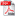# FINITE-DIFFERENCE APPROACH OF THE PHASECHANGE HEAT CONDUCTION IN A SQUARE-SHAPED DOMAIN

Autor/autori: Prof. Bogdan HORBANIUC, Prof. Gheorghe DUMITRASCU

Abstract: The paper approaches the study of solidification within a square domain by means of an implicit finite difference method. To simplify the mathematical treatment of the problem, we use the decomposition technique. The imposed boundary condition is of the third kind (known convection heat transfer coefficient). As the decomposition technique applied “as is” to the two-dimension conduction heat transfer with phase-change led to a distorted shape of the solid/liquid interface, we have developed an original approach, referred to as the "symmetry condition technique”. The numerical results show a good accuracy compared with other approaches.

Keywords: unsteady conduction heat transfer, phase-change, implicit finite difference technique, decomposition method.DOWNLOAD PDF